Next: The vector space model Up: Term frequency and weighting Previous: Inverse document frequency   Contents   Index

## Tf-idf weighting

We now combine the definitions of term frequency and inverse document frequency, to produce a composite weight for each term in each document. The tf-idf weighting scheme assigns to term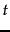a weight in document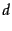given by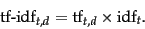(22)

In other words,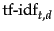assigns to terma weight in documentthat is

1. highest whenoccurs many times within a small number of documents (thus lending high discriminating power to those documents);

2. lower when the term occurs fewer times in a document, or occurs in many documents (thus offering a less pronounced relevance signal);

3. lowest when the term occurs in virtually all documents.

At this point, we may view each document as a vector with one component corresponding to each term in the dictionary, together with a weight for each component that is given by (22). For dictionary terms that do not occur in a document, this weight is zero. This vector form will prove to be crucial to scoring and ranking; we will develop these ideas in Section 6.3 . As a first step, we introduce the overlap score measure: the score of a documentis the sum, over all query terms, of the number of times each of the query terms occurs in. We can refine this idea so that we add up not the number of occurrences of each query termin, but instead the tf-idf weight of each term in.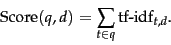(23)

In Section 6.3 we will develop a more rigorous form of Equation 23.

Exercises.

• Why is the idf of a term always finite?

• What is the idf of a term that occurs in every document? Compare this with the use of stop word lists.

• Consider the table of term frequencies for 3 documents denoted Doc1, Doc2, Doc3 in Figure 6.9 .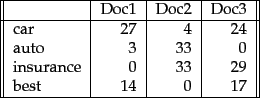Compute the tf-idf weights for the terms car, auto, insurance, best, for each document, using the idf values from Figure 6.8 .

• Can the tf-idf weight of a term in a document exceed 1?

• How does the base of the logarithm in (21) affect the score calculation in (23)? How does the base of the logarithm affect the relative scores of two documents on a given query?

• If the logarithm in (21) is computed base 2, suggest a simple approximation to the idf of a term.Next: The vector space model Up: Term frequency and weighting Previous: Inverse document frequency   Contents   Index